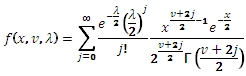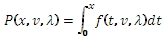# SQL Server non-central chi-squared inverse distribution function

CHI2NCINV

Updated: 31 July 2010

Use CHI2NC to calculate the inverse of the lower cumulative distribution function of the non-central chi-square distribution.

The probability density function is:The lower cumulative distribution function is:Syntax
SELECT [[wctStatistics].[wct].[CHI2NCINV] (
<@P, float,>
,<@DF, float,>
,<@Lambda, float,>)
Arguments
@P
is the cumulative distribution function. @P is an expression of type float or of a type that implicitly converts to float
@DF
is the degrees of freedom. @DF is an expression of type float or of a type that implicitly converts to float.
@Lambda
is the non-centrality parameter. @Lambda is an expression of type float or of a type that implicitly converts to float.
Return Types
float
Remarks
·         @DF must be greater than zero (@DF > 0).
·         @Lambda must be greater than or equal to zero (@Lambda ≥ 0).
·         @P must be greater than or equal to zero and less than or equal to one (0 ≤ @P ≤ 1).
Examples
Calculate the cumulative distribution function:
SELECT wct.CHI2NC(1,3,3)
This produces the following result
----------------------
0.0582469145317944

(1 row(s) affected)

Calculate the inverse:
SELECT wct.CHI2NCINV(0.0582469145317944,3,3)
This produces the following result
----------------------
1

(1 row(s) affected)

### SupportCopyright 2008-2021 Westclintech LLC         Privacy Policy        Terms of Service